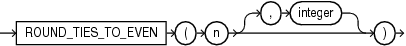ROUND_TIES_TO_EVEN (number)

Syntax

round_ties_to_even ::=Description of the illustration round_ties_to_even.eps

Purpose

ROUND_TIES_TO_EVEN is a rounding function that takes two parameters: n and integer. The function returns n rounded to integer places according to the following rules:

1. If integer is positive, n is rounded to integer places to the right of the decimal point.

2. If integer is not specified, then n is rounded to 0 places.

3. If integer is negative, then n is rounded to integer places to the left of the decimal point.

Restrictions

The function does not support the following types: BINARY_FLOAT and BINARY_DOUBLE.

Examples

The following example rounds a number to one decimal point to the right:

SELECT ROUND_TIES_TO_EVEN (0.05, 1) from DUAL

ROUND_TIES_TO_EVEN(0.05,1)
--------------------------
0

The following example rounds a number to one decimal point to the left:

SELECT ROUND_TIES_TO_EVEN(45.177,-1) "ROUND_EVEN" FROM DUAL;

ROUND_TIES_TO_EVEN(45.177,-1)
-----------------------------
50